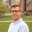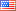Question-and-Answer Resource for the Building Energy Modeling Community
Get s tarted with the Help page

# Revision history [back]

### how to build and minimize the cost function using the output temperature(T) of a thermal system ?

if we suppose we have a thermal system for a single Room with two inputs,one for heat pump and the other for the heat of environment and then we Have T(temp) as an output of our system and I want to minimize the cost function having T ,How we should do it (the output)?

Thanks alot.2 retaggedMatthewSteen9749 ●13 ●25 https://www.nrel.gov/b...

### how to build and minimize the cost function using the output temperature(T) of a thermal system ?

if we suppose we have a thermal system for a single Room with two inputs,one for heat pump and the other for the heat of environment and then we Have T(temp) as an output of our system and I want to minimize the cost function having T ,How we should do it (the output)?

Thanks alot.3 retagged__AmirRoth__4388 ●5 ●16 http://bleedinggreenna...

### how to build and minimize the cost function using the output temperature(T) of a thermal system ?

if we suppose we have a thermal system for a single Room with two inputs,one for heat pump and the other for the heat of environment and then we Have T(temp) as an output of our system and I want to minimize the cost function having T ,How we should do it (the output)?

Thanks alot.